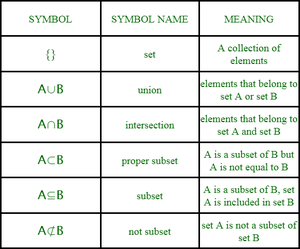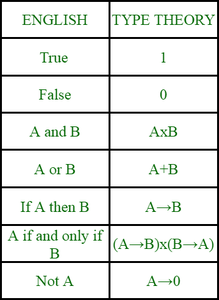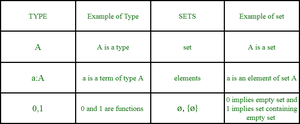Related Articles

# Semantic difference between Set and Type

• Last Updated : 28 Jul, 2021

Introduction :
Etymologically a set is a collection of distinct elements that may have something particular in common and type means-“of a particular kind”.

These two terms are vital in terms of mathematics as they give rise to set theory and type theory. This article provides semantic difference between set and type in terms of mathematics.

Attention reader! Don’t stop learning now. Get hold of all the important CS Theory concepts for SDE interviews with the CS Theory Course at a student-friendly price and become industry ready.

1. SET :
Overview of set –

• In mathematics set is a collection of distinct elements.
• A set is denoted by capital letters in italics such as A, E, D etc.
• A question asked in relation with set may result in true or false results.
• Sets lead to set theory.
• Set was founded in 19th century.
• George Cantor found set theory and is also known as father of set theory
• Set theory eventually leads to concept of lattices and then Boolean algebra. It is the root of Boolean algebra.Set theory

Ideology –

• The ideology of set is “collection”.
• Consider pre-defined objects in mathematics. These pre-defined objects can be analyzed, studied and can be put together in different groups based on certain factors. These groups form collection and a collection is a “set”.
• Example circle, points and line lie in a plane hence they can form a set.

Features of set –

• Sets have “elements” associated with it.
• In set theory an element can belong to more than one set.
• Numbers can be used to represent sets. ( 0 for empty set and 1 for a set containing an empty set).
• Set theory has its root from logic. Hence concept such as predicate logic need a separate system underneath it.
• Sets have operations associated with them. Some basic operations are- Unions, Intersection, Complement and Cartesian product.
• In order to check if an element belongs to a set, “proof” is required.

Applications of set –

• Set has its applications in mathematics. A set is universal (in terms of language) in mathematics. A set is used to construct relations.
• Set is used to identify and store unique elements in computer programming.
• Set lays the basics of foundation of Boolean algebra.
• Set lays the basics of a very important branch of computer science that is digital electronics.
• Set eventually led to the foundation of type theory.

Example –
N is a set of all natural numbers such that N={1,2,3,4,..} .Z is a set of all integers such that Z={-3,-2,-1,0,1,2,3,..}. A set B={true, false} is a set of Boolean values.

2. TYPE :Type theory

Overview of type –

• Type in mathematics is some kind of collection of values that are produced on evaluation of a term.
• A type is denoted by τ.
• In type one needs to proof whether question asked makes sense or not.
• Type leads to type theory.
• Type theory eventually led its foundation between 1902 and 1908 when Bertrand Russell proposed various theories of type.
• Type has its root from set theory.
• Type theory was made to eliminate inconsistencies of formal logics, rewrite system and naive set theory.

Ideology –

• The ideology of type is “construction”.
• In mathematics, objects are constructed according to rules.
• Organization of these objects on basis of their construction categorizes them into different “type”.
• Objects in mathematics are constructed in unique way resulting in unique types.

Features of type –

• Type has “terms” associated with it.
• In type theory terms generally belong to only one type.
• In type theory, numbers are used to represent functions. Terms  “and” and “or” can be encoded as type itself. No separate system is needed.
• Features associated with type are dependent types, equality types, inductive types, universe types and computational components.
• In order to check if a term is of particular type, “algorithm” is required.

Applications of type –

• Type theory creates practical impact on , proof assistants, programming languages, mathematical foundations, linguistics and social sciences.
• In programming languages type systems are use to identify bugs.
• Proof checkers, proof assistants, automated proof theorem use type theory to encode proofs in mathematics.
• Gregory Bateson logical level and  double bind notions are derived from type theory.
• Different types of grammar use type theory to categorize types (such as noun, verb).
• Type theory is used in semantics of language.

Examples –
If 3 is of type nat, then there exists a term of type I nat 3 3. 3+(7∗8)5   is also of type Nat. (In sets this can be represented by expressions 3∈{n∈N∣∀x,y,z∈N+(xn+yn≠zn)} ). M:A in type theory is evaluated as M is a term of data type A.Short  and basic examples

Conclusion –
Though sets and types are different yet they are  distinctly related. Each type gives rise to a set of entities of that type. In fact roots of type theory lie in set theory. Sets can also be regarded as type if considered in an extension. Different types may give rise to the same set.

My Personal Notes arrow_drop_up